Power of i(odd and even)

Chapter 5 Class 11 Complex Numbers
Concept wise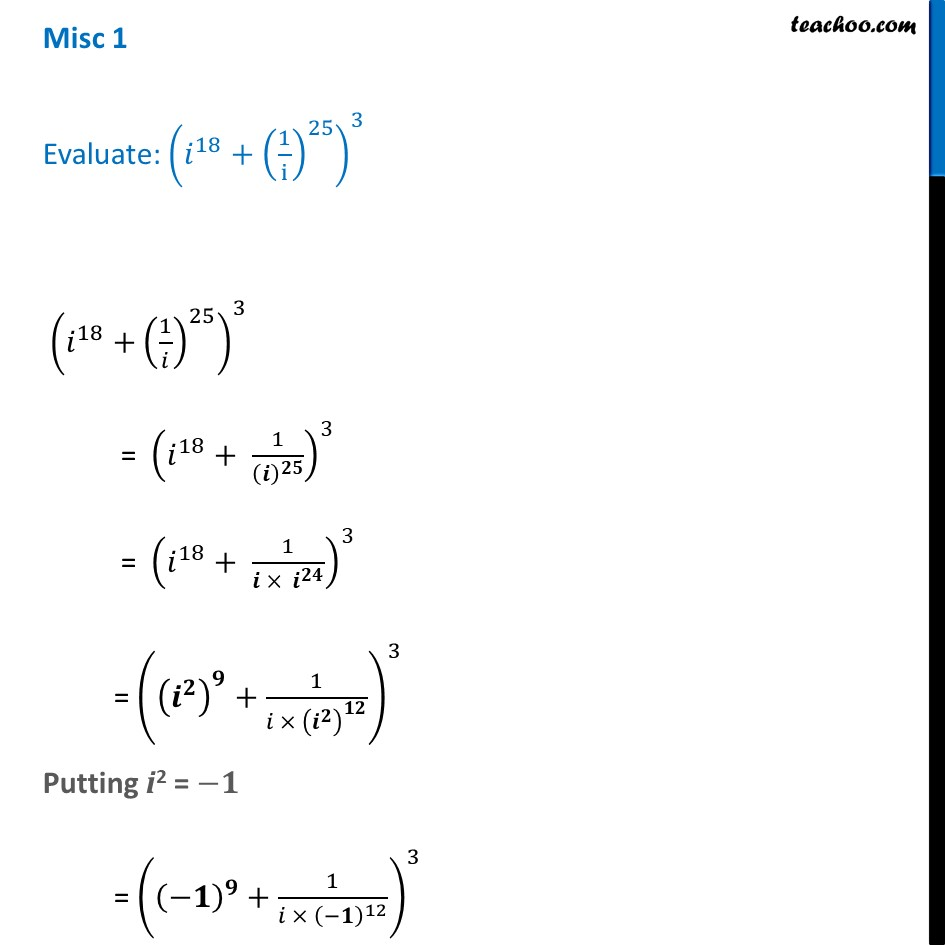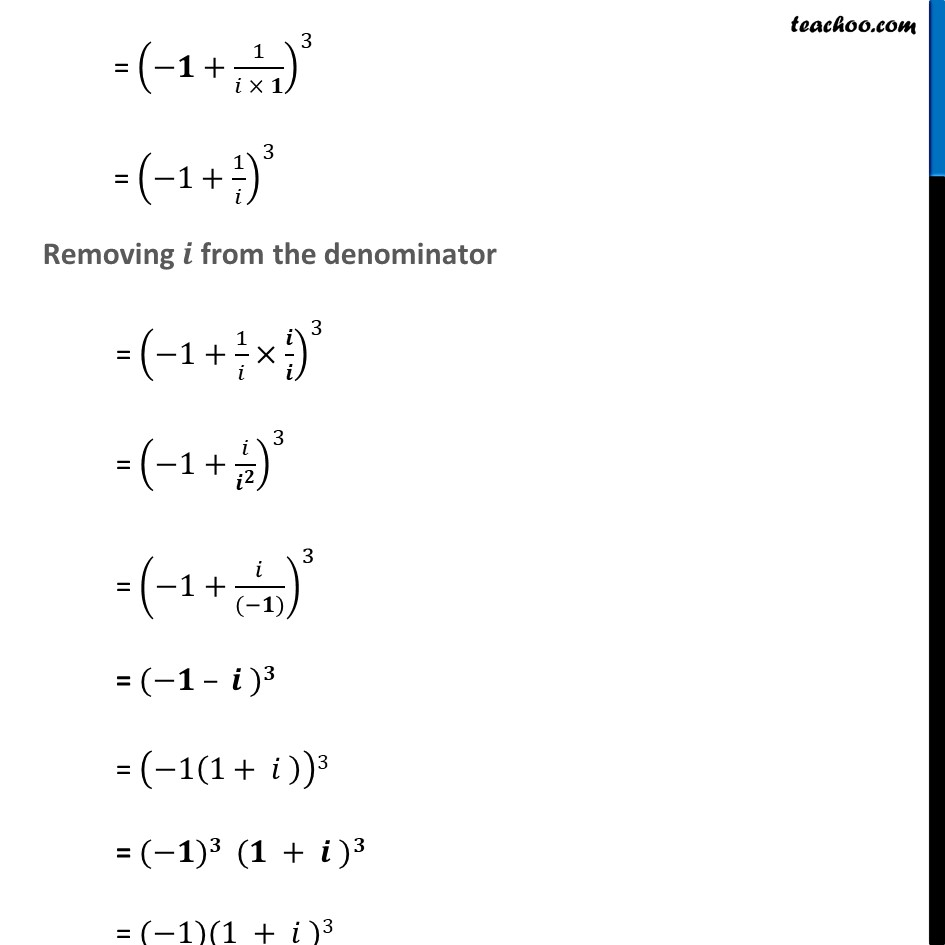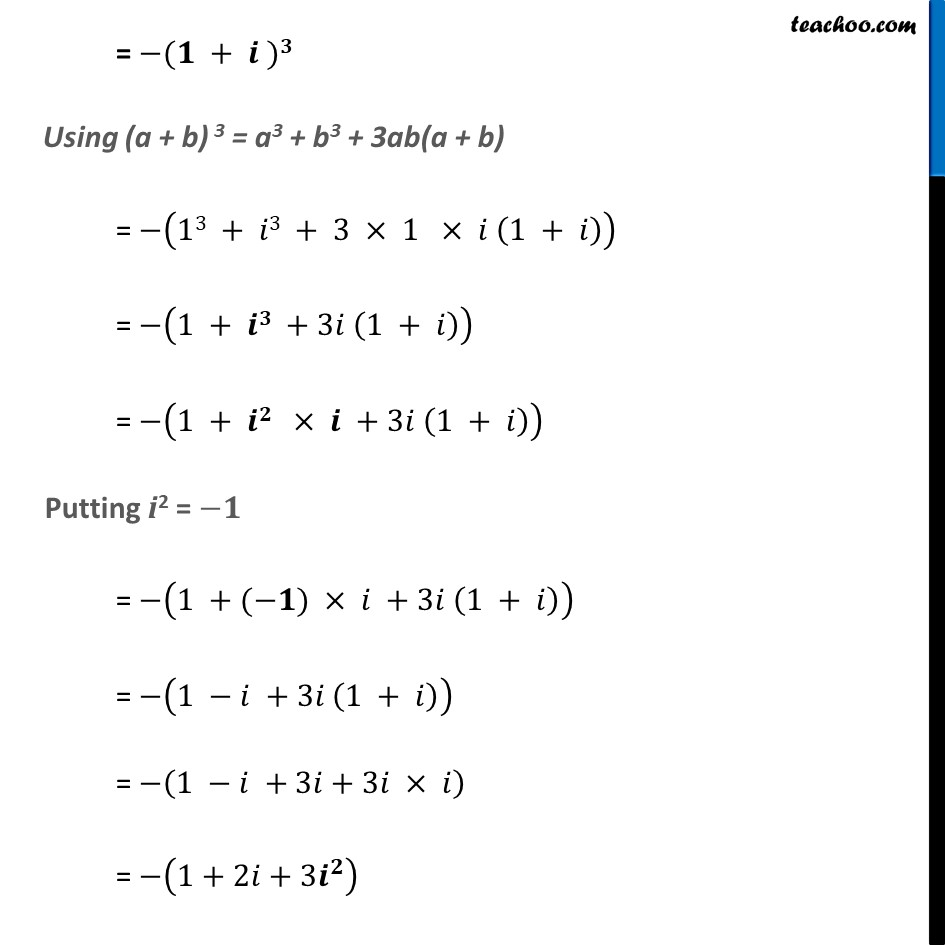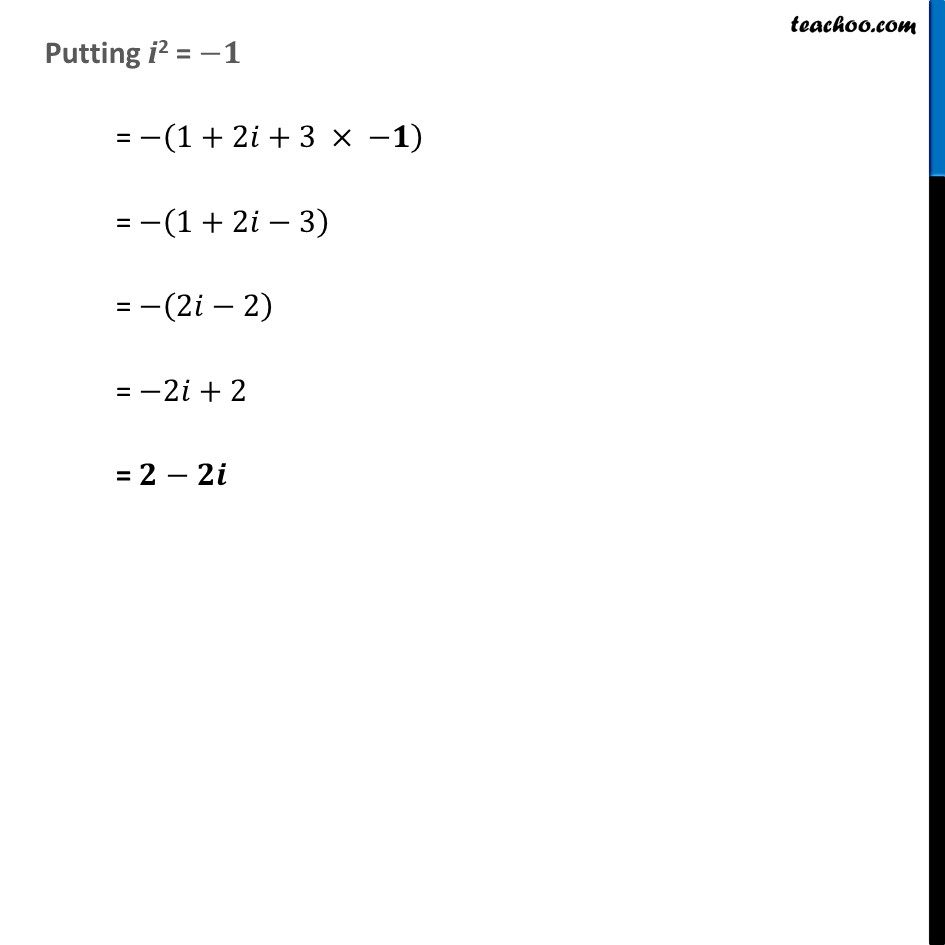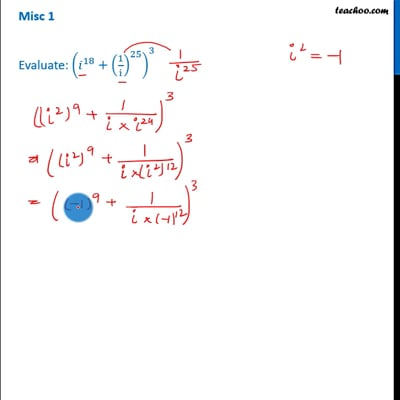This video is only available for Teachoo black users

Introducing your new favourite teacher - Teachoo Black, at only ₹83 per month

### Transcript

Misc 1 Evaluate: (𝑖^18+(1/i)^25 )^3 (𝑖^18+(1/𝑖)^25 )^3 = (𝑖^18+ 1/(𝒊)^𝟐𝟓 )^3 = (𝑖^18+ 1/(𝒊 × 𝒊^𝟐𝟒 ))^3 = ((𝒊^𝟐 )^𝟗+1/(𝑖 × (𝒊^𝟐 )^𝟏𝟐 ))^3 Putting i2 = −𝟏 = ((−𝟏)^𝟗+1/〖𝑖 × (−𝟏)〗^12 )^3 = (−𝟏+1/(𝑖 × 𝟏))^3 = (−1+1/𝑖)^3 Removing 𝒊 from the denominator = (−1+1/𝑖×𝒊/𝒊)^3 = (−1+𝑖/𝒊^𝟐 )^3 = (−1+𝑖/((−𝟏)))^3 = (−𝟏 – 𝒊 )𝟑 = (−1(1+ 𝑖 ))3 = (−𝟏)𝟑 (𝟏 + 𝒊 )𝟑 = (−1)(1 + 𝑖 )3 = −(𝟏 + 𝒊 )𝟑 Using (a + b) 3 = a3 + b3 + 3ab(a + b) = −(13 + 𝑖3 + 3 × 1 × 𝑖 (1 + 𝑖)) = −(1 + 𝒊𝟑 +3𝑖 (1 + 𝑖)) = −(1 + 𝒊𝟐 × 𝒊 +3𝑖 (1 + 𝑖)) Putting i2 = −𝟏 = −(1 +(−𝟏) × 𝑖 +3𝑖 (1 + 𝑖)) = −(1 −𝑖 +3𝑖 (1 + 𝑖)) = −(1 −𝑖 +3𝑖+3𝑖 × 𝑖) = −(1+2𝑖+3𝒊^𝟐 ) Putting i2 = −𝟏 = −(1+2𝑖+3 × −𝟏) = −(1+2𝑖−3) = −(2𝑖−2) = −2𝑖+2 = 𝟐−𝟐𝒊